# COVID-19 Rhythmanalysis

Rhythms permeate the city, and these rhythms overlap, combine, aggregate and interfere with one another. That’s the gist of Henri Lefebvre’s book entitled Rhythmanalysis. By my reading the concept fits within the genre of research concerned with everydayness, the quotidian, which implies a concern with ordinary things and everyday phenomena that repeat. (See post: Time and tide wait for no one.)The book first appeared in 1992 before the boom in big data. What computers could do with repetitions, cycles and rhythms was beyond ordinary.

Data that looks random often harbours regular patterns. As an example I examined the online data for COVID-19 cases picked up by testing in Scotland over the past 29 days. The data is available in spreadsheet format at https://www.gov.scot/publications/coronavirus-covid-19-trends-in-daily-data/ and looks like this

18 7 19 6 3 5 11 17 21 23 7 22 10 16 20 27 4 3 4 22 17 30 18 31 18 23 64 66 43

That’s the number of people shown up by tests each day. Here I graphed the numbers over the 29 days using the chart function on a spreadsheet.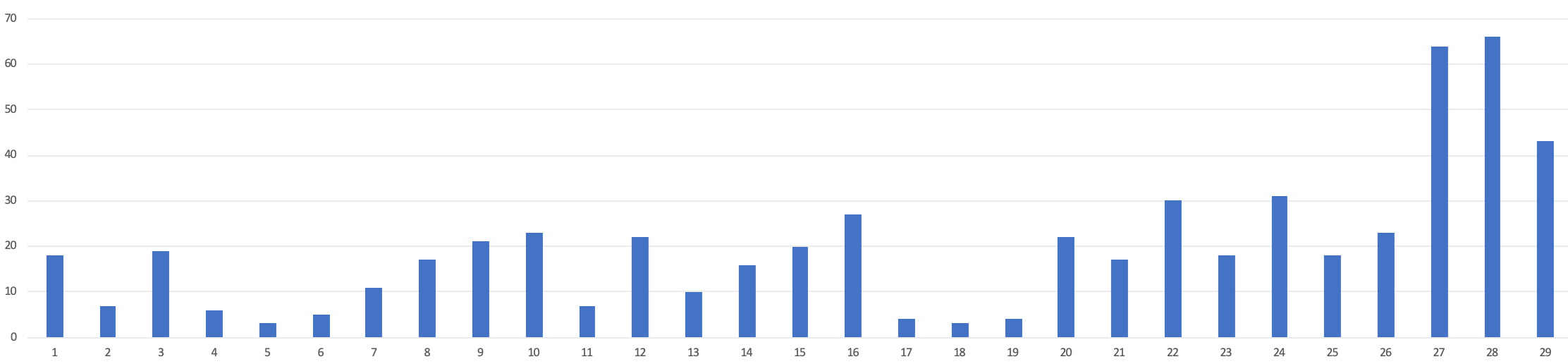It already looks as though there are cycles in play. Here’s the same data plotted as a smooth wavy line. I’ve moved the plot so that the midpoint between the maximum and minimum values lies on the horizontal axis. Oscillating graphs usually move about an axis. So this translation helps the calculation.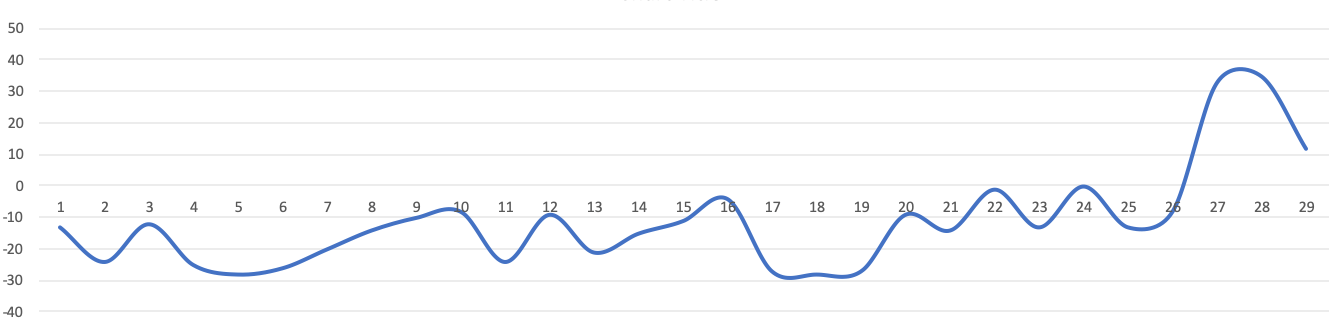### Where’s the beat?

The objective of this exercise is to find a series of cosine curves that average out to produce the same wavy line as the one above. Cosine curves are always uniform, and repeat in a regular manner. The wavy line above connecting the data points isn’t a cosine curve.

My previous post was about image compression and demonstrated the DCT (Discrete Cosine Transform) method with a line of up just 8 grey-scale pixels. The method derived the proportions of each of a sequence of standard cosine curves. In the 8-pixel exercise there were 8 candidate cosine curves. The more cosine curves the more likely you are to capture all the bumps and dips of the original data curve.

I extended the spreadsheet algorithm I used in my last post from 8 to 29 data points, and from 8 to 29 candidate cosine curves. The candidate cosine curves range from 0 to 15 cycles across the 29-day time span. Just so you know, those standard 29 unweighted cosine curves look something like this when placed on top of one another.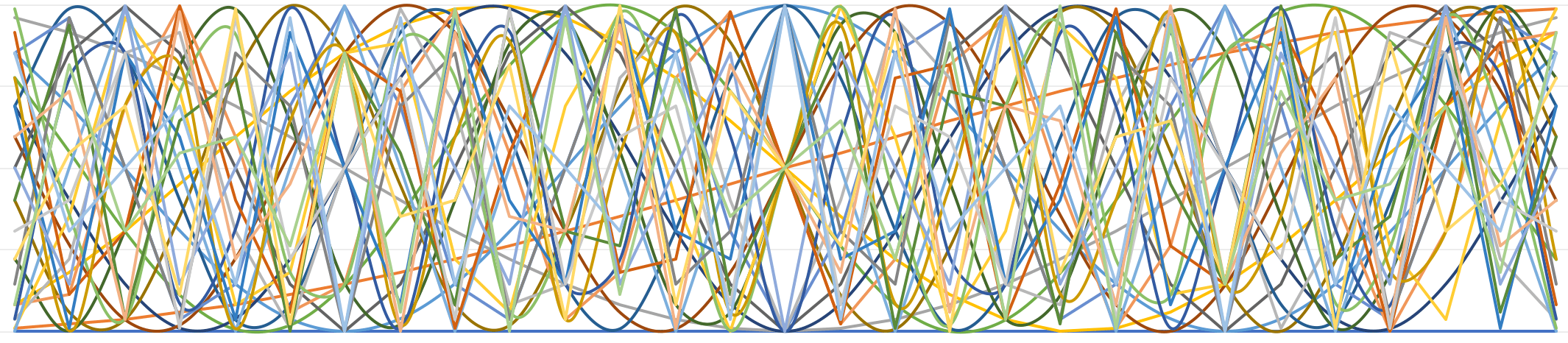Excel’s graph algorithms don’t use cosine smoothing, so the higher frequencies look a bit ragged in this chart. Here’s the spreadsheet showing the calculations using the DCT formula shown in my previous post. The result of this calculation is the column of coefficients second on the left showing the contribution of each frequency curve to the initial wavy line connecting the data points. The second row of the table heads each of the 1-29 days. To remind myself that this is real data, I coloured the days that fell on a Sunday in grey.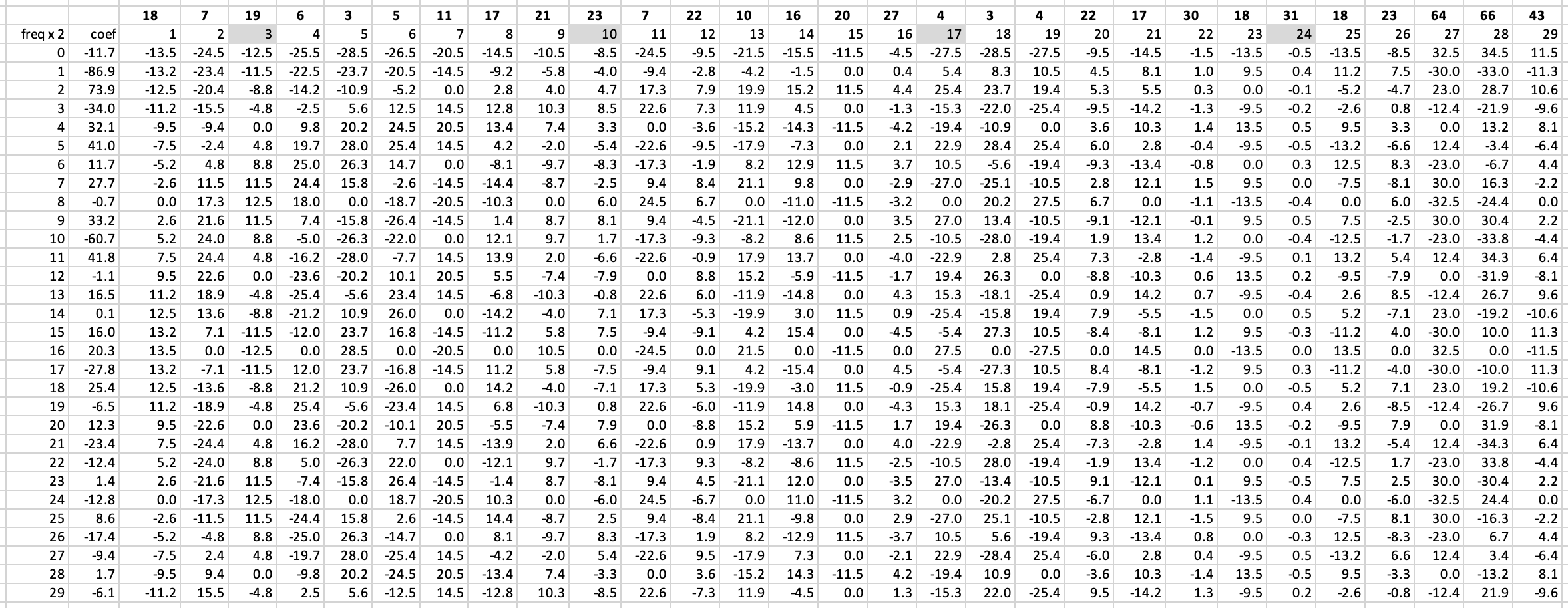When those coefficients are run through an inverse DCT algorithm the spreadsheet recomputes a plausible approximation of the original curvy line.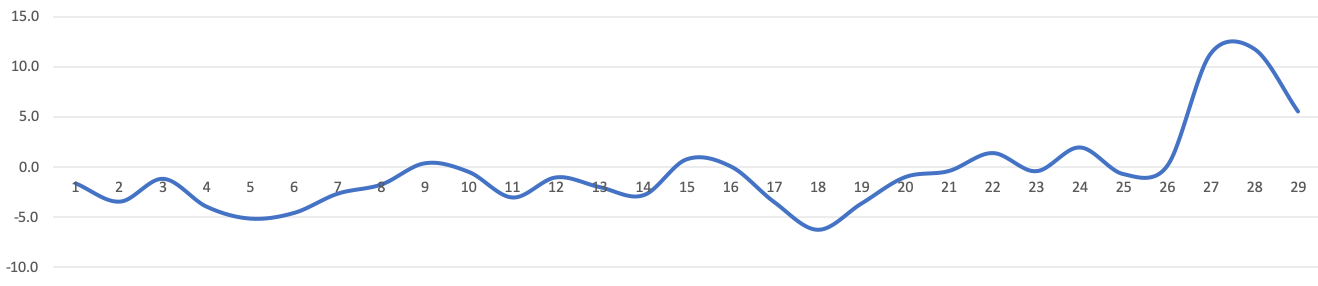That visualisation provides a good way of testing that the method of deriving the coefficients is working. To keep the calculation simple, I’ve left out various translation factors that would restore the original range of numbers in the data and that would move the new curve relative to the horizontal axis. Those factors are less important, as I really want to identify the most important cycles within the original data. The chart showing the contribution of each of the 29 cosine curves is a glorious mess, especially considering the limits of the spreadsheet chart smoothing algorithm. The black wavy line is the average of all those curves.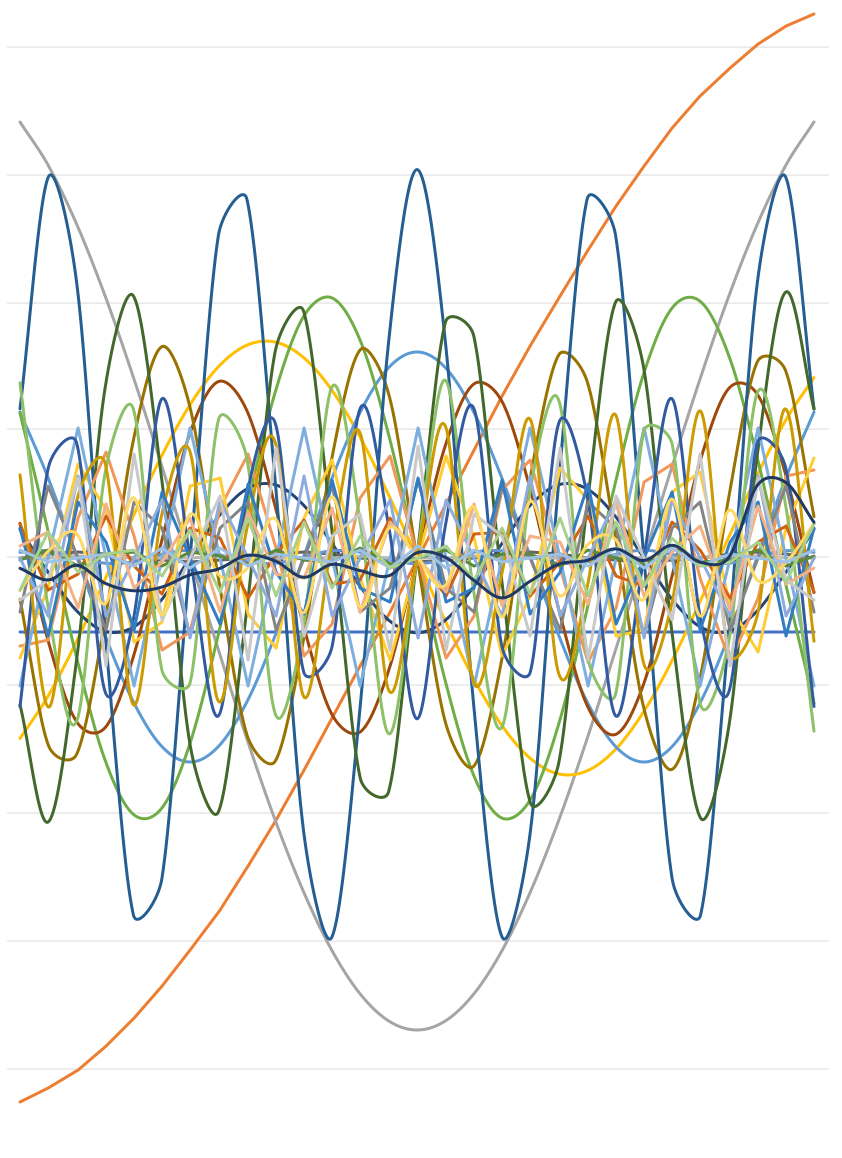### Filter out the little bumps

To extract anything useful from this tangle it’s necessary to filter out those cosine curves that make little contribution to the final curvy line. I set up an adjustable filter mechanism on the spreadsheet so that I could eliminate cosine curves that fell below a certain coefficient value. By trial and error I found that eliminating cosine curves with a coefficient less than 50 (plus or minus) gave the following simplified pattern.The contributing cosine curves (unweighted) are as follows.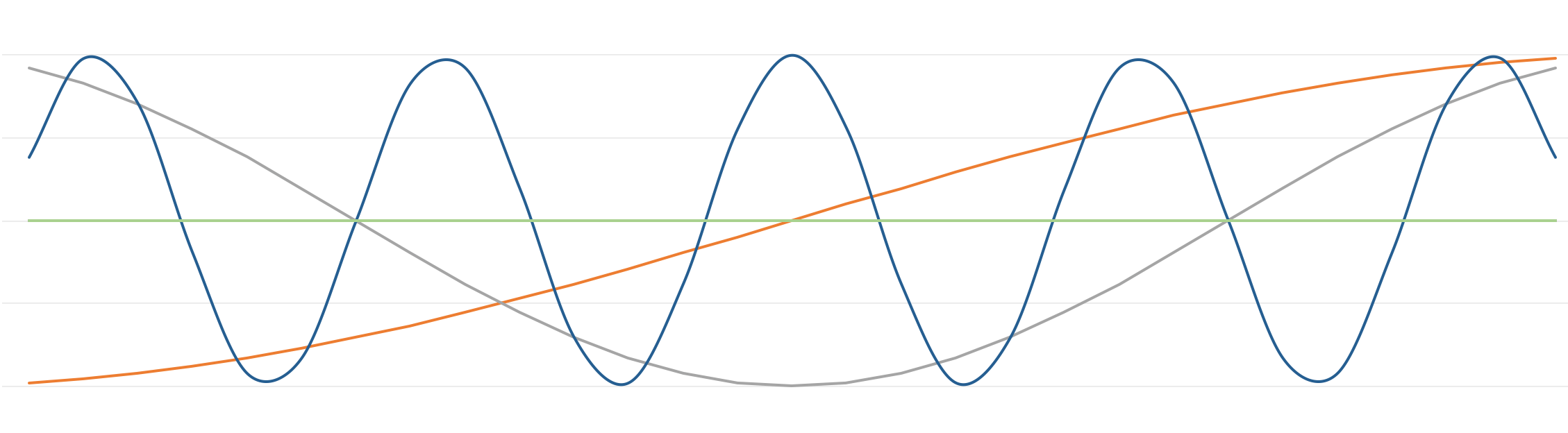So a data analyst might decide from this data manipulation that there was a drop in cases in the middle section of the month; the rises in data follow a regular cycle (perhaps weekly), and there’s a general increase over that period. Lowering the coefficient threshold to 40 revealed two more cycles.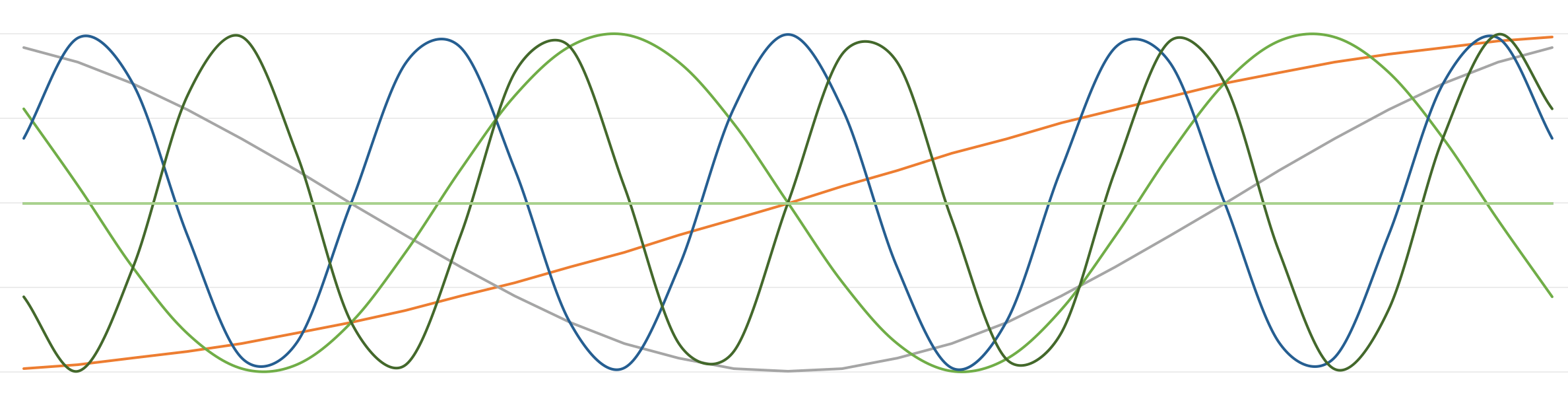This filtering process is analogous to what happens when DCT is used to filter out the high frequency components of a data sequence. I started to to carry out the same DCT analysis for a period longer than 29 days, but that required sampling the data every second day, or adding more coefficient calculations and the process started to get out of hand.

### Where do the rhythms come from?

It’s even more interesting to speculate on what contributes to the various bumps on the graph. It’s possible that parts of the data are random, or noisy due to the reporting mechanism or the nature of the phenomenon being recorded. Singular events may also defy any fit with a regular pattern.

Cyclical models are helpful however. You can think of singular, non-recurring events as occurring at an extremely low frequency over years, decades and centuries. Here are some possible contributors to the cycles.

• Organisational: testing and reporting on a weekly cycle; availability of testing: supply and delivery patterns, lab availability
• Human behavioural: effects of news cycles, public information campaigns, lockdowns, openings and other adjustments to human practices affecting people’s ability to present for a test or their susceptibility to the disease, including cyclical changes in diets, medication, drugs, holidays and travel.
• The overall upward trajectory probably indicates an increase in infections, as per the rate of transmission, i.e. the R number.
• Biological: alterations in the virus, waves of resistance, immunity, other diseases, other environmental responses of the organisms/virions, or the introduction of new vectors for transmission. I would expect that to require more than 29 days to show up.
• External: weather, tides, seasons, sun activity, etc. There are cycles here, but are they likely to show up?

I am just using the COVID data as an example to illustrate the DCT method of analysing urban data. I can’t claim any validity in the processing or outputs in social medical or epidemiological terms. I could have conducted this demonstration with the FTSE 100 index, or any other data that exhibits cyclical trends.

### References

• Ahmed, Nasir, T. Natarajan, and K. R. Rao. 1974. Discrete Cosine Transform. IEEE Transactions on Computers, (C-23)90–93.
• Lefebvre, Henri. 2004. Rhythmanalysis: Space, Time and Everyday Life. Trans. Stuart Elden, and Gerald Moore. London: Continuum

Notes

• The DCT method derives a coefficient value for any particular frequency by calculating the expected or idealised cosine value at that particular point on the horizontal axis (time in this case) multiplied by the actual data value. The results of these multiplications are then added together to give the coefficient for that cosine frequency. A higher value (plus or minus) coefficient indicates which frequencies contribute more to the original data sequence.
• If the numbers of cases are sampled, (e.g. once a week but not averaged) then the CDT method could be used to predict (i.e. interpolate the missing values). Prediction (i.e. extrapolation) beyond the range (in this case 29 days) is much less reliable as the method assumes the data values will oscillate, i.e. exhibit periodicity. Extrapolating the cosine curves above would miss out on growth in the data values beyond the range of the horizontal axis. For that kind of prediction, something like the exponential curve generated from a rate of infection would be more useful. See post Living with virions [and R0].
• Google searches foreground DCT as a filtering mechanism for image compression, but the original paper by Ahmed et al presents it as a means to recognising patterns in data: “the DCT can be used in the area of image processing for the purposes of feature selection in pattern recognition; and scalar-type Wiener filtering” (93)
• I tested my proposition above that the more cosine curves of increasing frequency you test against the original data, the more likely you are to capture all the bumps and dips of the original data curve. I tried this and it seemed not to add greater accuracy. The method seems to work best across a set of relatively smooth data, as seen across 8 pixels on a digital photo image. Hence, the applicability of DCT to image compression.